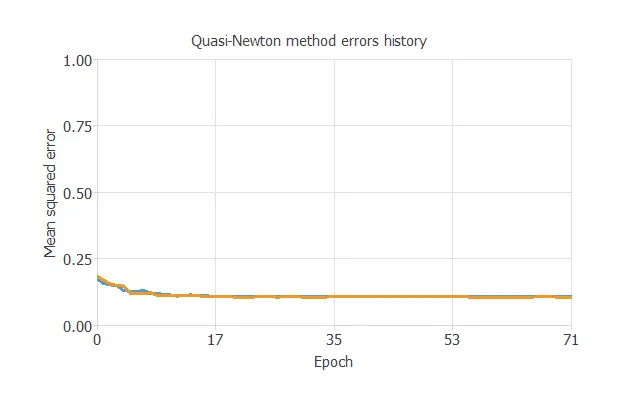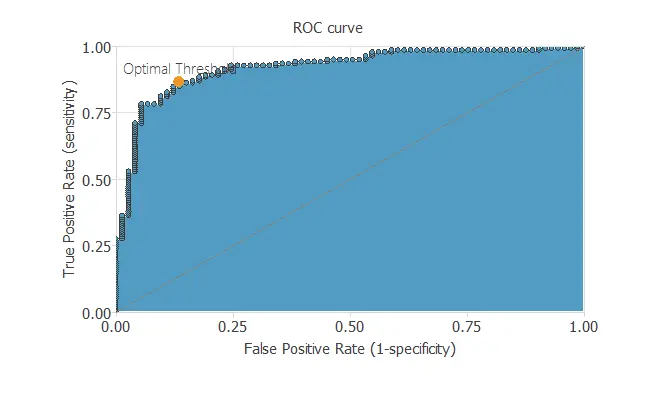# Predict biodegradability of different chemicals using machine learning

This example aims to catalog different chemicals depending on whether they are biodegradable or not.

QSAR (Quantitative Structure-Activity Relationships) models are currently being developed with more frequency nowadays.### Contents:

This example is solved with Neural Designer. To follow it step by step, you can use the free trial.

## 1. Application type

The variable to be predicted can have two values (ready or not ready biodegradable molecule). Thus, this is a binary classification project.

The goal here is to observe the correlation between different molecular descriptors with the biodegradability or not of a molecule.

## 2. Data set

The file biodegradation.csv contains 1055 samples of chemicals, each of them with 41 inputs and one of them is a binary target.

This data set contains the following variables:

• SpMax_L: Leading eingvalue from Laplace matrix.
• J_Dz(e): Balaban-like index from Barysz matrix weighted by Sanderson electronegativity.
• nHM: Number of heavy atoms.
• F01[N-N]: Frequency of N-N at topological distance 1.
• F04[C-N]: Frequency of C-N at topological distance 4.
• NssssC: Number of atoms of type ssssC.
• nCb: Number of substituted benzene C(sp2).
• C%: Percentage of C atoms.
• nCp: Number of terminal primary C(sp3).
• nO: Number of oxygen atoms.
• F03[C-N]: Frequency of C-N at topological distance 3.
• SdssC: Sum of dssC E-states.
• HyWi_B(m): Hyper-Wiener-like index (log function) from Burden matrix weighted by mass.
• LOC: Lopping centric index.
• SM6_L: Spectral moment of order 6 from Laplace matrix.
• F03[C-O]: Frequency of C - O at topological distance 3.
• Me: Mean atomic Sanderson electronegativity (scaled on Carbon atom).
• Mi: Mean first ionization potential (scaled on Carbon atom).
• nN-N: Number of N hydrazines.
• nArNO2: Number of nitro groups (aromatic).
• nCRX3: Number of CRX3.
• SpPosA_B(p): Normalized spectral positive sum from Burden matrix weighted by polarizability.
• nCIR: Number of circuits.
• B01[C-Br]: Presence/absence of C - Br at topological distance 1.
• B03[C-Cl]: Presence/absence of C - Cl at topological distance 3.
• N-073: Ar2NH / Ar3N / Ar2N-Al / R..N..R
• Psi_i_1d: Intrinsic state pseudoconnectivity index - type 1d.
• B04[C-Br]: Presence/absence of C - Br at topological distance 4.
• SdO: Sum of dO E-states.
• TI2_L: Second Mohar index from Laplace matrix.
• nCrt: Number of ring tertiary C(sp3.)
• C-026: R--CX--R.
• F02[C-N]: Frequency of C - N at topological distance 2.
• nHDon: Number of donor atoms for H-bonds (N and O).
• SpMax_B(m): Leading eigenvalue from Burden matrix weighted by mass.
• Psi_i_A: Intrinsic state pseudoconnectivity index - type S average.
• nN: Number of Nitrogen atoms.
• SM6_B(m): Spectral moment of order 6 from Burden matrix weighted by mass.
• nArCOOR: Number of esters (aromatic).
• nX: Number of halogen atoms.
• Mi: Mean first ionization potential (scaled on Carbon atom).

Finally, the use of all instances is set. Note that each instance contains a different chemical's input and target variables. The data set is divided into training, validation, and testing subsets. 60% of the instances will be assigned for training, 20% for generalization, and 20% for testing.

Once the data set has been set, we can perform a few related analytics. We check the provided information and make sure that the data has good quality.

We can calculate the data statistics and draw a table with the minimums, maximums, means, and standard deviations of all variables in the data set. The next table depicts the values.Also, we can calculate the distributions for all variables. The following figure shows a pie chart with the proportion of ready biodegradable (positives) and not ready biodegradable (negatives) chemicals in the data set.As we can see, the number of ready biodegradable chemicals is 33.7% of the samples, and not ready biodegradable represent 66.3% of the samples approximately.

Finally, the inputs-targets correlations might indicate to us what factors most influence in biodegradability.Here, the most correlated variables are SpMax_B(m), SM6_B(m), SpMax_L and SpMax_A, whose descriptions are above.

## 3. Neural network

The second step is to set a neural network to represent the classification function. For this class of applications, the neural network is composed of:

• Scaling layer.
• Perceptron layers.
• Probabilistic layer.

The scaling layer contains the statistics on the inputs calculated from the data file and the method for scaling the input variables. Here the minimum and maximum method has been set. Nevertheless, the mean and standard deviation method would produce very similar results.

The number of perceptron layers is 1. This perceptron layer has 41 inputs and 3 neurons.

Finally, we will set the binary probabilistic method for the probabilistic layer as we want the predicted target variable to be binary.

## 4. Training strategy

The procedure used to carry out the learning process is called a training strategy. The training strategy is applied to the neural network to obtain the best possible performance. The type of training is determined by how the adjustment of the parameters in the neural network takes place. This process is composed of two terms:

• A loss index.
• An optimization algorithm.

The loss index is the mean squared error with L2 regularization. This is the default loss index for binary classification applications.

The learning problem can be stated as finding a neural network that minimizes the loss index. That is, a neural network that fits the data set (error term) and does not oscillate (regularization term).

The optimization algorithm that we use is the quasi-Newton method. This is also the standard optimization algorithm for this type of problem.

The following chart shows how the error decreases with the iterations during the training process.The final training and selection errors are training error = 0.108 WSE and selection error = 0.105 WSE, respectively.

## 5. Model selection

The objective of model selection is to find the network architecture with the best generalization properties, that is, that which minimizes the error on the selected instances of the data set.

More specifically, we want to find a neural network with a selection error of less than 0.105 WSE, which is the value that we have achieved so far.

Order selection algorithms train several network architectures with a different number of neurons and select that with the smallest selection error.

The incremental order method starts with a small number of neurons and increases the complexity at each iteration.

## 6. Testing analysis

The objective of the testing analysis is to validate the generalization performance of the trained neural network. To validate a classification technique, we need to compare the values provided by this technique to the observed values. We can use the ROC curve as it is the standard testing method for binary classification projects.The following table contains the elements of the confusion matrix. This matrix shows the number of negative and positive predicted values versus the real ones.

Predicted positive Predicted negative
Real positive 59(28%) 14(6.6%)
Real negative 15(7.1%) 123(58.3%)

As we can see, the number of instances that the model can correctly predict is 182 (86.3%), while it misclassifies is 29 (13.7%). It should be noted that of all our chemicals data, most are not ready biodegradable.

The binary classification tests are parameters for measuring the performance of a classification problem with two classes:

• Classification accuracy (ratio of instances correctly classified): 86.3%
• Error rate (ratio of instances misclassified): 13.7%
• Sensitivity (ratio of real positive which are predicted positive): 86.3%
• Specificity (ratio of real negative which are predicted negative): 86.2%

## 7. Model deployment

Once the neural network's generalization performance has been tested, the neural network can be saved for future use in the so-called model deployment mode.

We can predict if a chemical molecule can be ready or not ready biodegradable calculating the neural network outputs. For that, we need to know the input variables for them. An example is the following:

We can export the mathematical expression of the neural network which take inputs variables to produce output variables, in our case, to decide the biodegradability. In classification models, the information is propagated feed-forward through the scaling, perceptron, ande probabilisticlayersr. This expression is listed below.

```scaled_J_Dz(e) = J_Dz(e)*(1+1)/(91.77500153-(0.8039000034))-0.8039000034*(1+1)/(91.77500153-0.8039000034)-1;
scaled_nHM = (nHM-(0.7165880203))/1.461760044;
scaled_F01[N-N] = (F01[N-N]-(0.04265400022))/0.2558889985;
scaled_F04[C-N] = (F04[C-N]-(0.9800950289))/2.331850052;
scaled_NssssC = (NssssC-(0.2900469899))/1.07325995;
scaled_C% = C%*(1+1)/(60.70000076-(0))-0*(1+1)/(60.70000076-0)-1;
scaled_nCp = (nCp-(1.376299977))/1.962589979;
scaled_nO = (nO-(1.803789973))/1.774590015;
scaled_F03[C-N] = (F03[C-N]-(1.436969995))/3.115099907;
scaled_SdssC = (SdssC-(-0.1971289963))/0.769298017;
scaled_LOC = (LOC-(1.350710034))/0.7857940197;
scaled_F03[C-O] = F03[C-O]*(1+1)/(40-(0))-0*(1+1)/(40-0)-1;
scaled_Me = Me*(1+1)/(1.31099999-(0.9570000172))-0.9570000172*(1+1)/(1.31099999-0.9570000172)-1;
scaled_Mi = Mi*(1+1)/(1.376999974-(1.021999955))-1.021999955*(1+1)/(1.376999974-1.021999955)-1;
scaled_nN-N = nN-N*(1+1)/(2-(0))-0*(1+1)/(2-0)-1;
scaled_nArNO2 = (nArNO2-(0.07393360138))/0.3173229992;
scaled_nCRX3 = (nCRX3-(0.02938389964))/0.2178940028;
scaled_nCIR = (nCIR-(1.405689955))/4.786270142;
scaled_B01[C-Br] = (B01[C-Br]-(0.03981040046))/0.1955129951;
scaled_B03[C-CI] = (B03[C-CI]-(0.1478669941))/0.3549689949;
scaled_N-073 = (N-073-(0.03127960116))/0.1994490027;
scaled_Psi_i_1d = Psi_i_1d*(1+1)/(1.072999954-(-1.098999977))+1.098999977*(1+1)/(1.072999954+1.098999977)-1;
scaled_B04[C-Br] = B04[C-Br]*(1+1)/(1-(0))-0*(1+1)/(1-0)-1;
scaled_SdO = SdO*(1+1)/(71.16699982-(0))-0*(1+1)/(71.16699982-0)-1;
scaled_TI2_L = TI2_L*(1+1)/(17.53700066-(0.4440000057))-0.4440000057*(1+1)/(17.53700066-0.4440000057)-1;
scaled_nCrt = (nCrt-(0.1298580021))/0.6437550187;
scaled_F02[C-N] = (F02[C-N]-(1.274880052))/2.272919893;
scaled_nHDon = (nHDon-(0.9611369967))/1.256420016;
scaled_Psi_i_A = (Psi_i_A-(2.558419943))/0.6424599886;
scaled_nN = (nN-(0.6862559915))/1.089869976;
scaled_nArCOOR = (nArCOOR-(0.05118479952))/0.3188149929;
scaled_nX = (nX-(0.723222971))/2.238219976;

perceptron_layer_0_output_0 = logistic[ -0.177432 + (scaled_J_Dz(e)*0.0855846)+ (scaled_nHM*-1.24226)+ (scaled_F01[N-N]*-0.283113)+ (scaled_F04[C-N]*-0.35339)+ (scaled_NssssC*-0.856399)+ (scaled_C%*-0.139905)+ (scaled_nCp*-0.289111)+ (scaled_nO*-0.20023)+ (scaled_F03[C-N]*-0.322568)+ (scaled_SdssC*0.132424)+ (scaled_LOC*0.360906)+ (scaled_F03[C-O]*-0.303221)+ (scaled_Me*0.154041)+ (scaled_Mi*0.187736)+ (scaled_nN-N*0.125482)+ (scaled_nArNO2*-0.448966)+ (scaled_nCRX3*-0.221001)+ (scaled_nCIR*-0.511291)+ (scaled_B01[C-Br]*-0.111304)+ (scaled_B03[C-CI]*-0.116656)+ (scaled_N-073*0.139527)+ (scaled_Psi_i_1d*-0.103048)+ (scaled_B04[C-Br]*0.176509)+ (scaled_SdO*0.540496)+ (scaled_TI2_L*0.414255)+ (scaled_nCrt*-0.302632)+ (scaled_F02[C-N]*-0.031898)+ (scaled_nHDon*-0.120617)+ (scaled_Psi_i_A*0.698333)+ (scaled_nN*-0.467465)+ (scaled_nArCOOR*0.454082)+ (scaled_nX*-0.336846) ];
perceptron_layer_0_output_1 = logistic[ 0.190828 + (scaled_J_Dz(e)*-0.106789)+ (scaled_nHM*1.33593)+ (scaled_F01[N-N]*0.309528)+ (scaled_F04[C-N]*0.369096)+ (scaled_NssssC*0.920219)+ (scaled_C%*0.146187)+ (scaled_nCp*0.320681)+ (scaled_nO*0.299069)+ (scaled_F03[C-N]*0.339289)+ (scaled_SdssC*-0.148324)+ (scaled_LOC*-0.407062)+ (scaled_F03[C-O]*0.345641)+ (scaled_Me*-0.172198)+ (scaled_Mi*-0.203953)+ (scaled_nN-N*-0.133797)+ (scaled_nArNO2*0.463928)+ (scaled_nCRX3*0.241401)+ (scaled_nCIR*0.564473)+ (scaled_B01[C-Br]*0.105944)+ (scaled_B03[C-CI]*0.109147)+ (scaled_N-073*-0.151173)+ (scaled_Psi_i_1d*0.118023)+ (scaled_B04[C-Br]*-0.19303)+ (scaled_SdO*-0.585528)+ (scaled_TI2_L*-0.428076)+ (scaled_nCrt*0.309396)+ (scaled_F02[C-N]*0.0309882)+ (scaled_nHDon*0.121517)+ (scaled_Psi_i_A*-0.760547)+ (scaled_nN*0.496675)+ (scaled_nArCOOR*-0.487881)+ (scaled_nX*0.353319) ];

pprobabilistic_layer_combinations_0 = -0.0876607 +2.58938*perceptron_layer_0_output_0 -2.83917*perceptron_layer_0_output_1

RB_NRB = 1.0/(1.0 + exp(-probabilistic_layer_combinations_0);

logistic(x){
return 1/(1+exp(-x))
}
```

We have developed an algorithm that classifies the biodegradability of a molecule for different chemicals.

## References:

• The data for this problem has been taken from the UCI Machine Learning Repository.
• Mansouri, K., Ringsted, T., Ballabio, D., Todeschini, R., Consonni, V. (2013). Quantitative Structure - Activity Relationship models for ready biodegradability of chemicals. Journal of Chemical Information and Modeling, 53, 867-878.